# 网络流建模方法一览

​ 还有一些dalao的博客，不过也都只是因为不想打字了就copy一些很通俗的结论过来，全文几乎都是笔者的原创。

## 一、傻逼建图法

（注：笔者选取了洛谷为题目来源，原因竟是视觉效果友好）


1.每个点最多被选中一次
2.某些点对之间有必选关系

## 七、动态思想

### 3.经典的例子

#### [NOI2012]美食节&&[SCOI2007]修车

##### 数据范围

(2<=M<=9,1<=N<=60), (1<=T<=1000)

##### Solution

$W_n\times 1+W_{n-1} \times 2+...+W_1 \times n$

##### 题目描述 2.0

m个厨师都会制作全部的n种菜品，但对于同一菜品，不同厨师的制作时间未必相同。他将菜品用1, 2, ..., n依次编号，厨师用1, 2, ..., m依次编号，将第j个厨师制作第i种菜品的时间记为 ti,j 。小M认为：每个同学的等待时间为所有厨师开始做菜起，到自己那份菜品完成为止的时间总长度。换句话说，如果一个同学点的菜是某个厨师做的第k道菜，则他的等待时间就是这个厨师制作前k道菜的时间之和。而总等待时间为所有同学的等待时间之和。现在，小M找到了所有同学的点菜信息: 有 pi 个同学点了第i种菜品（i=1, 2, ..., n）。他想知道的是最小的总等待时间是多少。

## 八、二分图

### 3.最小覆盖数=最大匹配数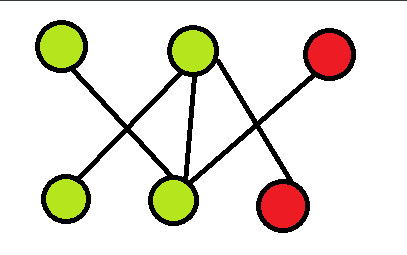### 4. 最大独立集=总点数-最小覆盖集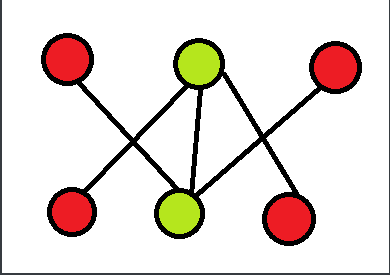## 九、经典的建模

### 3.数字梯形问题

①从梯形的顶至底的 m 条路径互不相交；

②从梯形的顶至底的 m 条路径仅在数字结点处相交；

③从梯形的顶至底的 m 条路径允许在数字结点相交或边相交。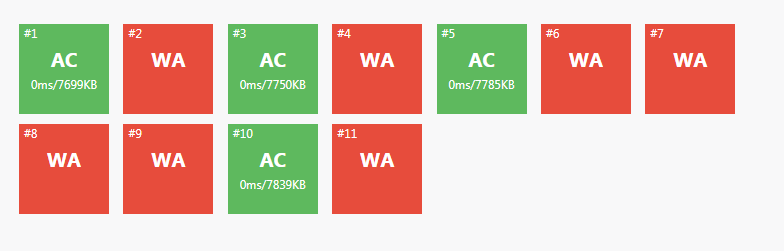### 8.最大密度子图

$g(\lambda)=max(|E|-\lambda|V|)$

g(λ)=0时我们取到最优解。

$g(\lambda)=max\lbrace a(x)-\lambda b(x)\rbrace$

$g(\lambda)<0⇔\lambda>\lambda^*$

$g(\lambda)=0⇔ \lambda=\lambda^*$

$g(\lambda)>0⇔ \lambda<\lambda^*$

### 12.混合图的欧拉回路

#### 12.1欧拉回路

(1)给定一个图G，假设我们能一笔画，如果图G中的一个路径包括每个边恰好一次，则该路径称为欧拉路径(Euler path)。如果一个回路是欧拉路径，则称为欧拉回路(Euler circuit)。

(2)对于一个无向图，如果每个点的度数均为偶数，那么这个图是一个欧拉图。

(3)对于一个有向图，如果每个点的出度都等于入度，那么这个图是一个欧拉图。

(4)对于无向图，如果每个点度数均为偶数，或者有且仅有两个顶点度数为奇数，那么这个图中存在欧拉路径。

(5)对于有向图，如果每个点的出度等于入度，或者有且仅有两个点不符，且这两个点一个入度比出度小1，一个出度比入度小1，则这个图存在欧拉路径。

#### 12.3求解混合欧拉图

所谓的自调整方法就是将其中的一些边的方向调整回来，使所有的点的出度等于入度。但是有一条边的方向改变后，可能会改变一个点的出度的同时改变另一个点的入度，相当于一条边制约着两个点。同时有些点的出度大于入度，迫切希望它的某些点出边转向；而有些点的入度大于出度，迫切希望它的某些入边转向。这两条边虽然需求不同，但是他们之间往往一条边转向就能同时满足二者。


#### 12.4算法步骤

1.$首先对于每个入度>出度的点，我们将其与源点S连接一条权值为\frac{入度-出度}{2}的边；$

$对于每个入度<出度的点，我们将其与汇点T连接一条权值为\frac{出度-入度}{2}的边。$

2.然后将原图中所有你定了向的无向边连接的两个点连一条边权为1，方向为你定向方向的无向边。

3.跑网络流去，如果满流自然就有解。

4.把在网络流中那些因为原图无向边而建的流量为1的边中经过流量的边反向，就形成了一个能跑出欧拉回路的有向图，如果要求方案，用有向图求欧拉回路的方法求解即可 。

### 13.最大权闭合子图

#### 13.3并不感性的证明

$最小割=|X_0|+|Y_1|$

$Sum_X+最小割=X_1-|X_0|+|X_0|+Y_1$

$Sum_X+最小割=X_1+Y_1=原图所有正权点权值之和$

## 十、几道脑洞大开的题目

### 1.[SDOI2017]新生舞会

#### 题面描述

Cathy收集了这些同学之间的关系，比如两个人之前认识没计算得出 $a_{i,j}$

Cathy还需要考虑两个人一起跳舞是否方便，比如身高体重差别会不会太大，计算得出 $b_{i,j}$ ，表示第i个男生和第j个女生一起跳舞时的不协调程度。

Cathy想先用一个程序通过$a_{i,j}$$b_{i,j}$ 求出一种方案，再手动对方案进行微调。

Cathy找到你，希望你帮她写那个程序。

C=$\frac{a'_1+a'_2+...+a'_n}{b'_1+b'_2+...+b'_n}′$

Cathy希望C值最大。

#### Solution

#include<iostream>
#include<cstdio>
#include<cstring>
#include<cmath>
#include<algorithm>
#include<queue>
#define inf 1000000000000001ll
#define maxxx 500000001
#define re register
#define ll long long
#define min(a,b) a<b?a:b
using namespace std;
const long double eps=0.00000007;
struct po{
int to,nxt,w;
ll dis;
};
po edge;
int n,m,s,t,b,p;
ll tot,dis,pa,pb;
{
int x=0,c=1;
char ch=' ';
while((ch>'9'||ch<'0')&&ch!='-')ch=getchar();
while(ch=='-')c*=-1,ch=getchar();
while(ch<='9'&&ch>='0')x=x*10+ch-'0',ch=getchar();
return x*c;
}
inline void add_edge(int from,int to,int w,ll dis)
{
edge[num].to=to;
edge[num].w=w;
edge[num].dis=dis;
}
inline void add(int from,int to,int w,ll dis)
{
}
inline bool spfa()
{
for(re int i=s;i<=t;i++) dis[i]=inf+1;
memset(b,0,sizeof(b));
queue<int> q;
q.push(t);
dis[t]=0;
b[t]=1;
while(!q.empty()){
int u=q.front();
q.pop();
b[u]=0;
int v=edge[i].to;
if(edge[i^1].w>0&&dis[v]>dis[u]-edge[i].dis){
dis[v]=dis[u]-edge[i].dis;
if(!b[v]){
b[v]=1;
q.push(v);
}
}
}
}
return dis[s]<inf;
}
inline int dfs(int u,int low)
{
b[u]=1;
if(u==t) return low;
int diss=0;
int v=edge[i].to;
if(edge[i].w&&!b[v]&&dis[v]==dis[u]-edge[i].dis){
int check=dfs(v,min(edge[i].w,low));
if(check){
tot+=check*edge[i].dis;
low-=check;
diss+=check;
edge[i].w-=check;
edge[i^1].w+=check;
if(low==0) break;
}
}
}
return diss;
}
inline void max_flow()
{
int ans=0;
while(spfa()){
b[t]=1;
while(b[t]){
memset(b,0,sizeof(b));
ans+=dfs(s,maxxx);

}
}
return;
}
inline void build(ll x)
{
num=-1;tot=0;
for(re int i=1;i<=n;i++)
for(re int i=1;i<=n;i++)
for(re int j=1;j<=n;j++)
}
inline bool check(ll x)
{
build(x);
max_flow();
if(-tot<=0) return 1;
else return 0;
}
int main()
{
for(re int i=1;i<=n;i++)
for(re int j=1;j<=n;j++)
for(re int i=1;i<=n;i++)
for(re int j=1;j<=n;j++)
s=0,t=n+n+1;
ll l=1,r=50000000000ll;
while(r>=l){
ll mid=(l+r)/2;
if(check(mid))
r=mid-1; else
l=mid+1;
}
printf("%.6lf",l*1.0/5000000);
}


### 2.[2018多省省队联测]劈配

#### 题目描述

（特别地，如果第i名没有填写志愿表、或其所有志愿中的导师战队均已满员，那么该选手出局）。#### 数据范围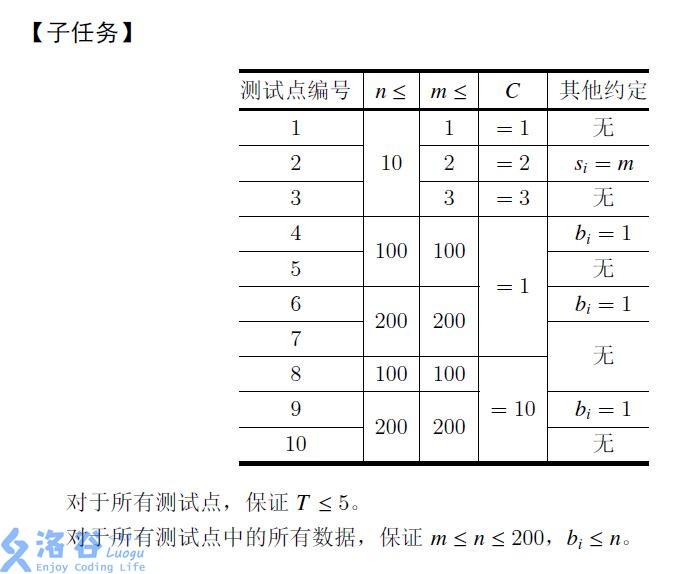#### Solution

##### 2.4正解算法

#include<iostream>
#include<cstring>
#include<cstdio>
#include<cmath>
#include<algorithm>
#include<queue>
#define re register
#define inf 50000001
#define ll long long
#define min(a,b) a<b?a:b
#define max(a,b) a>b?a:b
using namespace std;
struct po{
int nxt,to,w;
};
po edge;
int T,C,n,m,s,t;
int a,rs,ql,ans,cur,last,tag;
int out1,out2;
{
int x=0,c=1;
char ch=' ';
while((ch>'9'||ch<'0')&&ch!='-')ch=getchar();
while(ch=='-')c*=-1,ch=getchar();
while(ch<='9'&&ch>='0')x=x*10+ch-'0',ch=getchar();
return x*c;
}
inline void add_edge(int from,int to,int w)
{
edge[num].to=to;
edge[num].w=w;
}
inline void add(int from,int to,int w)
{
}
inline bool bfs()
{
memset(dep,0,sizeof(dep));
queue<int> q;
while(!q.empty())
q.pop();
q.push(s);
dep[s]=1;
while(!q.empty())
{
int u=q.front();
q.pop();
{
int v=edge[i].to;
if(dep[v]==0&&edge[i].w>0)
{
dep[v]=dep[u]+1;
if(v==t)
return 1;
q.push(v);
}
}
}
return 0;
}
inline int dfs(int u,int dis)
{
if(u==t) return dis;
int diss=0;
for(re int& i=cur[u];i!=-1;i=edge[i].nxt){
int v=edge[i].to;
if(edge[i].w!=0&&dep[v]==dep[u]+1){
int check=dfs(v,min(dis,edge[i].w));
if(check>0)
{
dis-=check;
diss+=check;
edge[i].w-=check;
edge[i^1].w+=check;
if(dis==0) break;
}
}
}
return diss;
}
inline void dinic()
{
while(bfs())
{
for(re int i=s;i<=t;i++)
while(int d=dfs(s,inf)) ans+=d;
}
}
void init(){
memset(out1,0,sizeof(out1));
memset(out2,0,sizeof(out2));
s=0;t=m+n+1;
for(re int i=1;i<=n;i++) out1[i]=m+1;
for(re int i=1;i<=n;i++)
for(re int j=1;j<=m;j++)
}
int main()
{
//freopen("date.in","r",stdin);
while(T--){
init();
for(re int i=1;i<=n;i++){
for(re int j=1;j<i;j++)
for(re int l=1;l<=m;l++)
dinic();
for(re int l=1;l<=m;l++){
for(re int j=1;j<=m;j++)
dinic();
if(edge[tag].w==0){out1[i]=l;break;}
}
}
for(re int i=1;i<=n;i++){
if(out1[i]<=want[i]) continue;
int l=1,r=i-1;out2[i]=i;
while(l<=r){
int mid=l+r>>1;
for(re int j=1;j<mid;j++)
for(re int l=1;l<=m;l++)
dinic();
for(re int j=1;j<=m;j++)
dinic();
if(edge[tag].w==0) {l=mid+1;out2[i]=i-mid;}
else r=mid-1;
}
}
for(re int i=1;i<=n;i++)
cout<<out1[i]<<" ";
cout<<endl;
for(re int i=1;i<=n;i++)
cout<<out2[i]<<" ";
cout<<endl;
}
}


### 3.[2017国家集训队测试]无限之环

#### 题目描述

，所有水管的粗细都相同，所以如果两个相邻方格的公共边界的中点都有接头，那么可以看作这两个接头互相连接
。水管有以下15种形状：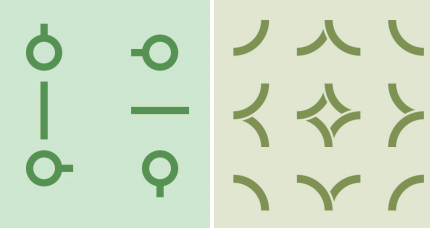#### 数据范围

$n×m≤2000$

#### Solution

##### 3.4回到题目中

#pragma comment(linker, "/STACK:1024000000,1024000000")
#include<iostream>
#include<cstdio>
#include<cstring>
#include<cmath>
#include<algorithm>
#include<queue>
#define inf 1000000001
#define re register
#define ll long long
#define min(a,b) a<b?a:b
#define MAXN 20001
#define MAXM 40000005
#define id1 nm[i][j]
#define id2 (nm[i][j]+(n*m))
#define id3 (nm[i][j]+(n*m*2))
#define id4 (nm[i][j]+(n*m*3))
#define id5 (nm[i][j]+(n*m*4))
using namespace std;
int n,m,s,t;
int to,nxt,w,edis;
int a,nm;
{
int x=0,c=1;
char ch=' ';
while((ch>'9'||ch<'0')&&ch!='-')ch=getchar();
while(ch=='-')c*=-1,ch=getchar();
while(ch<='9'&&ch>='0')x=x*10+ch-'0',ch=getchar();
return x*c;
}
inline void add_edge(int from,int too,int ww,ll dis)
{
to[num]=too;
w[num]=ww;
edis[num]=dis;
}
inline void add(int from,int too,int ww,ll dis)
{
}
inline bool spfa()
{
for(re int i=s;i<=t;i++) dis[i]=inf+1;
memset(b,0,sizeof(b));
deque<int> q;
q.push_back(t);
dis[t]=0;
b[t]=1;
while(!q.empty()){
int u=q.front();
q.pop_front();
b[u]=0;
int v=to[i];
if(w[i^1]>0&&dis[v]>dis[u]-edis[i]){
dis[v]=dis[u]-edis[i];
if(!b[v]){
b[v]=1;
if(!q.empty()&&dis[v]<dis[q.front()])
q.push_front(v);
else
q.push_back(v);
}
}
}
}
return dis[s]<inf;
}
inline int dfs(int u,int low)
{
b[u]=1;
if(u==t) return low;
int diss=0;
int v=to[i];
if(w[i]&&!b[v]&&dis[v]==dis[u]-edis[i]){
int check=dfs(v,min(w[i],low));
if(check){
tot+=check*edis[i];
low-=check;
diss+=check;
w[i]-=check;
w[i^1]+=check;
if(low==0) break;
}
}
}
return diss;
}
inline int max_flow()
{
int ans=0;
while(spfa()){
b[t]=1;
while(b[t]){
memset(b,0,sizeof(b));
ans+=dfs(s,inf);
}
}
return ans;
}
int main()
{
//freopen("date.in","r",stdin);
for(re int i=1;i<=n;i++)
for(re int j=1;j<=m;j++)
s=0;t=5*n*m+1;
for(re int i=1;i<=n;i++)
for(re int j=1;j<=m;j++){
}
for(re int i=1;i<=n;i++){
for(re int j=1;j<=m;j++)
if((i+j)%2==0){
} else {
}
}
for(re int i=1;i<=n;i++)
for(re int j=1;j<=m;j++){
if((i+j)%2==0){
}
}
int d=max_flow();
cout<<((maxx==d<<1)?tot:-1);
return 0;
}


### 4.[HAOI2017]新型城市化

#### 题目描述

Anihc国有n座城市.城市之间存在若一些贸易合作关系.如果城市x与城市y之间存在贸易协定.那么城市文和城市y则是一对贸易伙伴(注意:(x,y)和(y,x))是同一对城市)。

Anihc国需要在下一次会议上讨论扩大建设新型城市关系的问题.所以要请你求出在哪些城市之间建立贸易伙伴关系可以使得这个条件成立.即建立此关系前后的最大城市群的 大小至少相差1。

#### 输入输出样例

5 3
1 5
2 4
2 5


2
1 5
2 4


#### Code

#pragma comment(linker, "/STACK:1024000000,1024000000")
#include <iostream>
#include <cstdlib>
#include <cmath>
#include <string>
#include <cstring>
#include <algorithm>
#include <cstdio>
#include <queue>
#include <set>
#include <map>
#define MAXN 200000
#define re register
using namespace std;
typedef long long ll;
typedef unsigned long long ull;
#define ms(arr) memset(arr, 0, sizeof(arr))
const int inf = 0x3f3f3f3f;
struct po
{
int nxt,to,w,from;
}edge;
struct ANS
{
int x,y;
}ans[MAXN];
{
int x=0,c=1;
char ch=' ';
while((ch>'9'||ch<'0')&&ch!='-')ch=getchar();
while(ch=='-') c*=-1,ch=getchar();
while(ch<='9'&&ch>='0')x=x*10+ch-'0',ch=getchar();
return x*c;
}
inline void add_edge(int from,int to,int w)
{
edge[num].from=from;
edge[num].to=to;
edge[num].w=w;
}
inline void add(int from,int to,int w)
{
}
inline bool bfs()
{
memset(dep,0,sizeof(dep));
queue<int> q;
while(!q.empty())
q.pop();
q.push(s);
dep[s]=1;
while(!q.empty())
{
int u=q.front();
q.pop();
{
int v=edge[i].to;
if(dep[v]==0&&edge[i].w>0)
{
dep[v]=dep[u]+1;
if(v==t)
return 1;
q.push(v);
}
}
}
return 0;
}
inline int dfs(int u,int dis)
{
if(u==t)
return dis;
int diss=0;
for(re int& i=cur[u];i!=-1;i=edge[i].nxt)
{
int v=edge[i].to;
if(edge[i].w!=0&&dep[v]==dep[u]+1)
{
int check=dfs(v,min(dis,edge[i].w));
if(check!=0)
{
dis-=check;
diss+=check;
edge[i].w-=check;
edge[i^1].w+=check;
if(dis==0) break;
}
}
}
return diss;
}
inline void dinic()
{
while(bfs())
{
for(re int i=s;i<=t;i++)
while(int d=dfs(s,inf));
}
}
void put_color(int u,int col)
{
c[u]=col;
vis[u]=1;
int v=edge[i].to;
if(!vis[v]) put_color(v,col^1);
}
}
int dfn[MAXN],low[MAXN],stack[MAXN],color_num,color[MAXN],cnt,top;
inline void Tarjan(int u)
{
dfn[u]=low[u]=++cnt;
vis[u]=1;
stack[++top]=u;
if(!edge[i].w){
int v=edge[i].to;
if(!dfn[v]){
Tarjan(v);
low[u]=min(low[u],low[v]);
} else if(vis[v]) low[u]=min(low[u],dfn[v]);
}
}
if(dfn[u]==low[u]){
color[u]=++color_num;
vis[u]=0;
while(stack[top]!=u){
color[stack[top]]=color_num;
vis[stack[top--]]=0;
}
top--;
}
}
int x[MAXN],y[MAXN],tot;
inline bool cmp(ANS a,ANS b){
return a.x==b.x?a.y<b.y:a.x<b.x;
}
int main()
{
for(re int i=1;i<=m;i++){
}

for(re int i=1;i<=n;i++)
if(!vis[i]) put_color(i,2);
s=0,t=n+1;num=-1;
for(re int i=1;i<=n;i++){
if(c[i]==2)
}
for(re int i=1;i<=m;i++){
if(c[x[i]]==2)
}
dinic();
memset(vis,0,sizeof(0));
for(re int i=1;i<=n;i++)
if(!dfn[i]) Tarjan(i);
for(re int i=0;i<=num;i+=2){
int u=edge[i].from,v=edge[i].to;
if(!edge[i].w&&color[u]!=color[v]&&u!=s&&v!=t&&u!=t&&v!=s){
if(u>v) swap(u,v);
ans[++tot].x=u;ans[tot].y=v;
}
}

sort(ans+1,ans+tot+1,cmp);
cout<<tot<<endl;
for(re int i=1;i<=tot;i++)
printf("%d %d\n", ans[i].x,ans[i].y);
return 0;
}
/*6 5 3 7 2 4 1*/


posted @ 2018-03-13 19:18  ~victorique~  阅读(12402)  评论(15编辑  收藏  举报
Live2D